# 平面几何数学基础

## 1. 三角函数

### 1.1 三角函数基础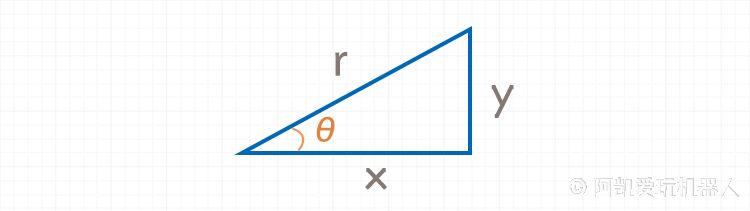### 1.2 正切与反正切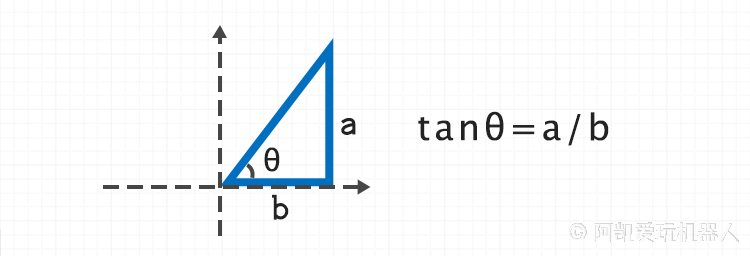### 1.3 arctan2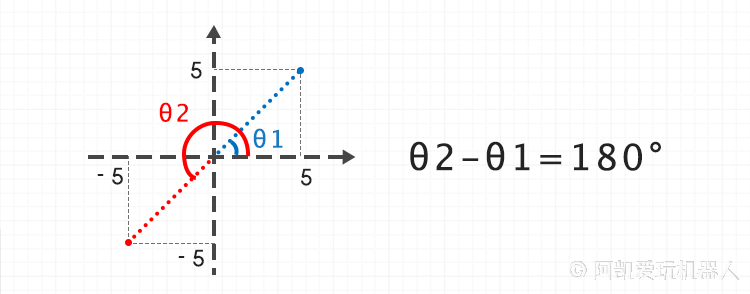### 1.4 已知AB求夹角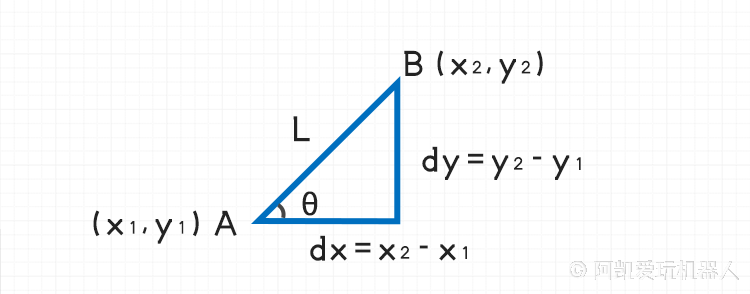$AB$ 直线与 $x$ 轴的夹角

### 1.5 已知一点、直线和夹角，求另一点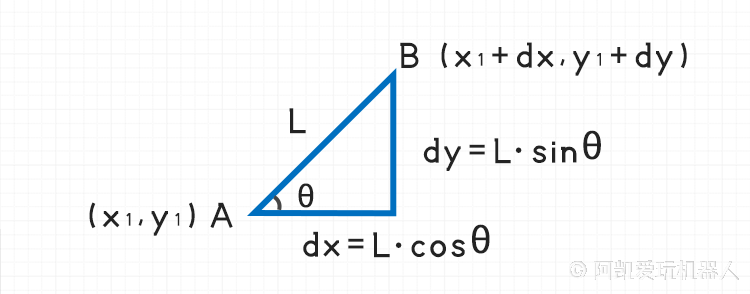### 1.6 余弦公式 - 已知三边求夹角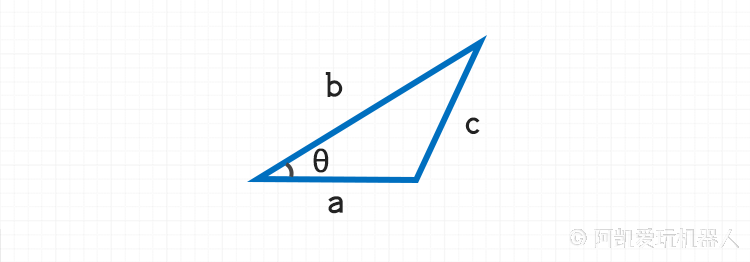import math
def cal_tri_angle(a, b, c):
'''返回边a与边b之间的夹角，弧度制'''
try:
theta = math.acos((a**2 + b**2 - c**2) / (2 * a * b))
except ValueError as e:
print(e)
print('a={}, b={}, c={}'.format(a, b, c))
exit(-1)
return theta


## 2. 向量

### 2.1 向量叉乘 (叉积)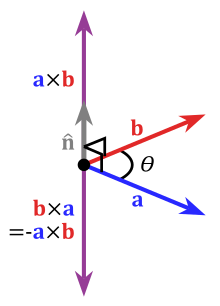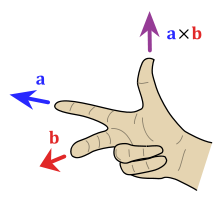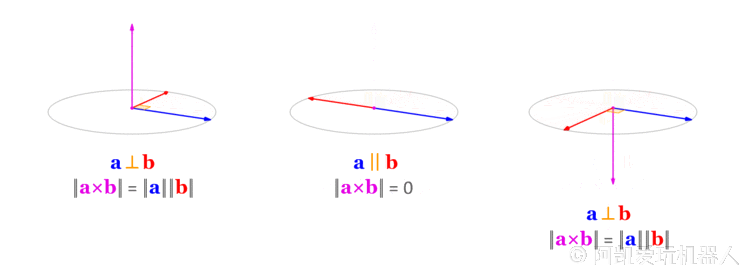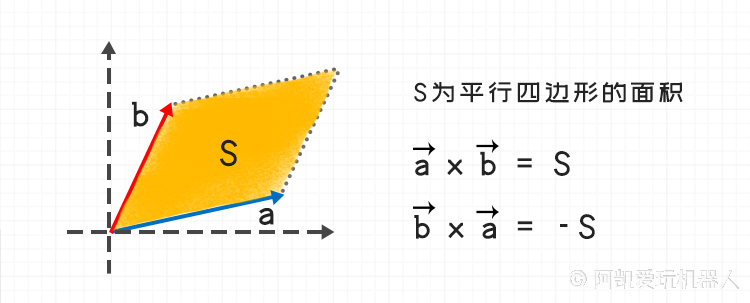Python代码片段如下：

def vector_cross_product(x1, y1, x2, y2):
'''向量叉乘'''
return x1 * y2 - x2 * y1

def is_vector_ccw(x1, y1, x2, y2):
'''向量a是否是逆时针旋转到向量b'''
return vector_cross_product(x1, y1, x2, y2) > 0


## 3. 圆

### 3.1 圆的表达式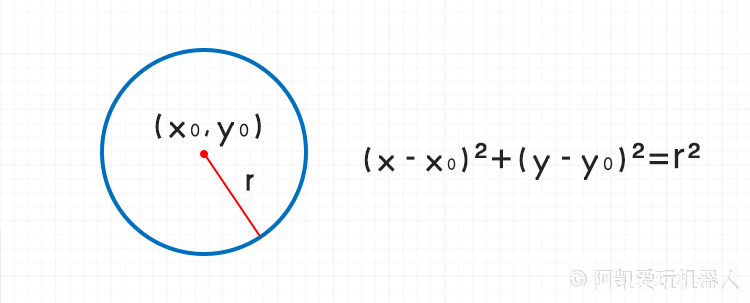### 3.2 两个圆求交点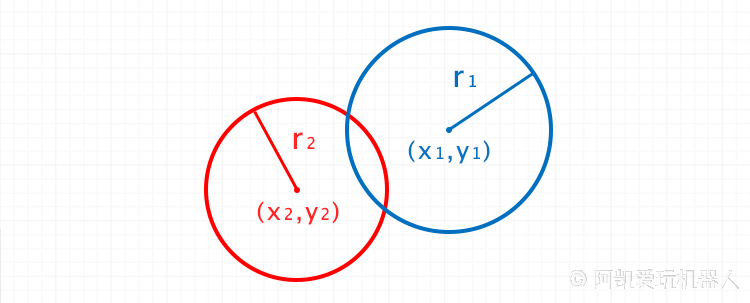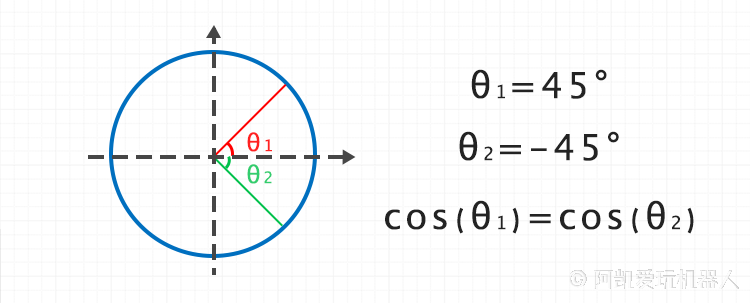$\theta$ 带入圆1的参数化方程里面，这里拿 $\theta_1$ 举例：

import math

def circle_inter_posi(x1, y1, r1, x2, y2, r2):
'''
圆之间的交叉点
'''
distance = math.sqrt((x1 - x2)**2 + (y1 - y2)**2)

if distance > (r1 + r2) or (x1 == x2 and y1 == y2):
print('Error: circle has not intersect points')
return False, None
else:
pass
# print('distance: {}'.format(distance))
a = 2 * r1 * (x1 - x2)
b = 2 * r1 * (y1 - y2)
c = (r2**2 - r1**2) - (x1 - x2)**2 - (y1 - y2)**2

p = a**2 + b**2
q = -2 * a * c
r = c**2 - b**2

cos_theta_1 = (-q + math.sqrt(q**2 - 4*p*r))/ (2 * p)
cos_theta_2 = (-q - math.sqrt(q**2 - 4*p*r))/ (2 * p)

# 存放所有可能的角度
angle_list = []
if abs(cos_theta_1) < 1:
angle_list.append(math.acos(cos_theta_1))
if abs(cos_theta_2) < 1:
angle_list.append(math.acos(cos_theta_2))
# 把角度负值也添加到angle_list中
for i in range(len(angle_list)):
theta = angle_list[i]
if theta == 0.0:
# 如果角度为0则不需要反转，因为-0跟0是同一个角度
continue
angle_list.append(-1*theta)

angle_list = list(set(angle_list))
# 存放交点
result = []
# 遍历验证角度是否正确
for angle in angle_list:
ix = x1 + r1 * math.cos(angle)
iy = y1 + r1 * math.sin(angle)

if abs(math.sqrt((ix-x2)**2 + (iy-y2)**2)-r2) <= 1e-3:
result.append((ix, iy))

return True, result